# Class 8 RD Sharma Solutions – Chapter 18 Practical Geometry – Exercise 18.2

• Last Updated : 05 May, 2021

### Question 1: Construct a quadrilateral ABCD in which AB = 3.8 cm, BC = 3.0 cm, AD = 2.3 cm, AC = 4.5 cm and BD = 3.8 cm?

Solution:

We have, AB = 3.8 cm, BC = 3.0 cm, AD = 2.3 cm, AC = 4.5 cm and BD = 3.8 cm.

Hey! Looking for some great resources suitable for young ones? You've come to the right place. Check out our self-paced courses designed for students of grades I-XII

Start with topics like Python, HTML, ML, and learn to make some games and apps all with the help of our expertly designed content! So students worry no more, because GeeksforGeeks School is now here!

Step 1: Draw a line segment AC = 6cm.

Step 2: Taking A as the centre, draw an arc of radius = 3.8 cm. Mark that point as B.

Step 3: Taking C as the centre, draw an arc of radius = 3 cm intersecting point B.

Step 4: Taking B as the centre, draw an arc of radius = 3.8 cm. Mark that point as D.

Step 5: Taking A as the centre, draw an arc of radius = 2.3 cm intersecting point D.

Step 6: Now join AB, BD, AD, and DC to form a quadrilateral.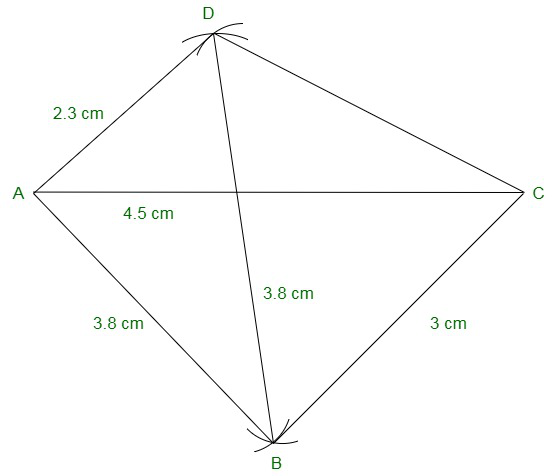### Question 2: Construct a quadrilateral ABCD in which BC = 7.5 cm, AC = AD = 6 cm, CD = 5 cm and BD = 10 cm?

Solution:

We have, BC = 7.5 cm, AC = AD = 6 cm, CD = 5 cm and BD = 10 cm.

Step 1: Draw a line segment AC = 6cm.

Step 2: Taking A as the centre, draw an arc of radius = 6 cm. Mark that point as D.

Step 3: Taking C as the centre, draw an arc of radius = 5 cm intersecting point D.

Step 4: Taking D as the centre, draw an arc of radius = 10 cm. Mark that point as B.

Step 5: Taking C as the centre, draw an arc of radius = 7.5 cm intersecting point B.

Step 6: Now join AD, CD, DB, and AB to form a quadrilateral.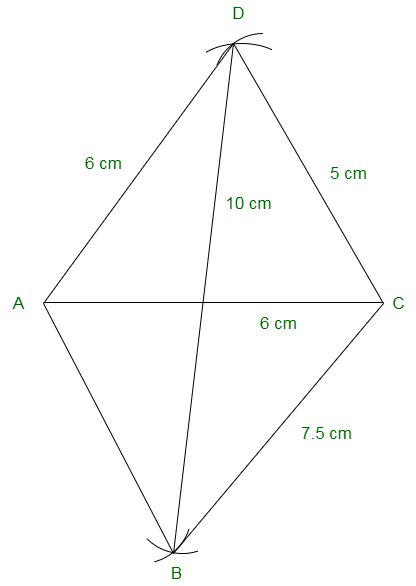### Question 3: Construct a quadrilateral ABCD when AB = 3 cm, CD = 3 cm, DA = 7.5 cm, AC = 8 cm and BD = 4 cm?

Solution:

We have, AB = 3 cm, CD = 3 cm, DA = 7.5 cm, AC = 8 cm and BD = 4 cm.

Consider a triangle ABD,

Now, AB + BD = 3 + 4 = 7 cm

Note: Sum of lengths of two sides of a triangle is always greater than the third side.

∴ The construction is not possible.

### Question 4: Construct a quadrilateral ABCD given AD = 3.5 cm, BC = 2.5 cm, CD = 4.1 cm, AC = 7.3 cm and BD = 3.2 cm?

Solution:

We have, AD = 3.5 cm, BC = 2.5 cm, CD = 4.1 cm, AC = 7.3 cm and BD = 3.2 cm.

Step 1: Draw a line segment CD = 4.1cm

Step 2: Taking C as the centre, draw an arc of radius = 7.3 cm. Mark that point as A.

Step 3: Taking D as the centre, draw an arc of radius = 3.5 cm intersecting point A.

Step 4: Taking D as the centre, draw an arc of radius = 3.2 cm. Mark that point as B.

Step 5: Taking C as the centre, draw an arc of radius = 2.5 cm intersecting point B.

Step 6: Now join CA, DA, DB, CB, and AB to form a quadrilateral.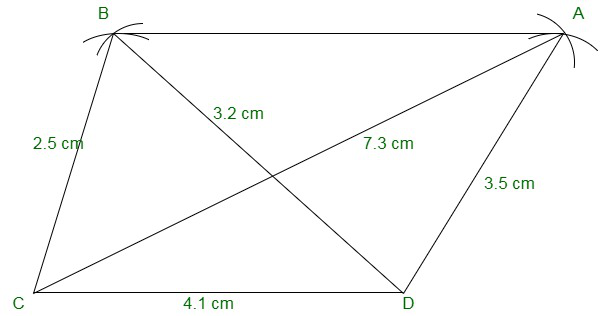### Question 5: Construct a quadrilateral ABCD given AD = 5 cm, AB = 5.5 cm, BC = 2.5 cm, AC = 7.1 cm and BD = 8 cm?

Solution:

We have, AD = 5 cm, AB = 5.5 cm, BC = 2.5 cm, AC = 7.1 cm and BD = 8 cm.

Step 1: Draw a line segment AB = 5.5 cm

Step 2: Taking B as the centre, draw an arc of radius = 2.5 cm. Mark that point as C.

Step 3: Taking A as the centre, draw an arc of radius = 7.1 cm intersecting point C.

Step 4: Taking B as the centre, draw an arc of radius = 8 cm. Mark that point as D.

Step 5: Taking A as the centre, draw an arc of radius = 5 cm intersecting point D.

Step 6: Join the line segments BC, AC, BD, AD, and CD to form a quadrilateral.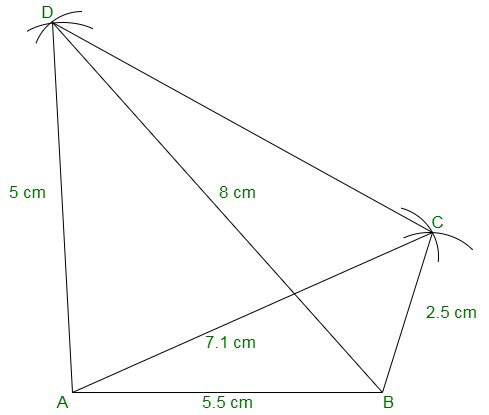### Question 6: Construct a quadrilateral ABCD in which BC = 4 cm, CA = 5.6 cm, AD = 4.5 cm, CD = 5 cm and BD = 6.5 cm?

Solution:

We have, BC = 4 cm, CA = 5.6 cm, AD = 4.5 cm, CD = 5 cm and BD = 6.5 cm.

Step 1: Draw a line segment BC = 4cm

Step 2: Taking B as the centre, draw an arc of radius = 6.5 cm. Mark that point as D.

Step 3: Taking C as the centre, draw an arc of radius = 5 cm intersecting point D.

Step 4: Taking C as the centre, draw an arc of radius = 5.6 cm. Mark that point as A.

Step 5: Taking D as the centre, draw an arc of radius = 4.5 cm intersecting point A.

Step 6: Join the line segments BD, CD, CA, DA, and AB to form a quadrilateral.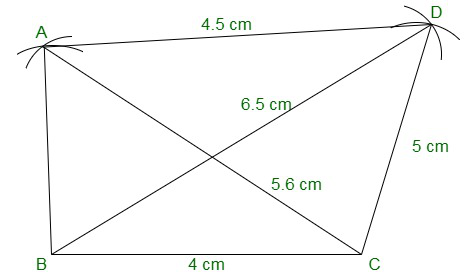My Personal Notes arrow_drop_up